Скачать презентацию LING 364 Introduction to Formal Semantics Lecture 17

8ecfd25fa33ea4ab2ec07071d020d63a.ppt

• Количество слайдов: 17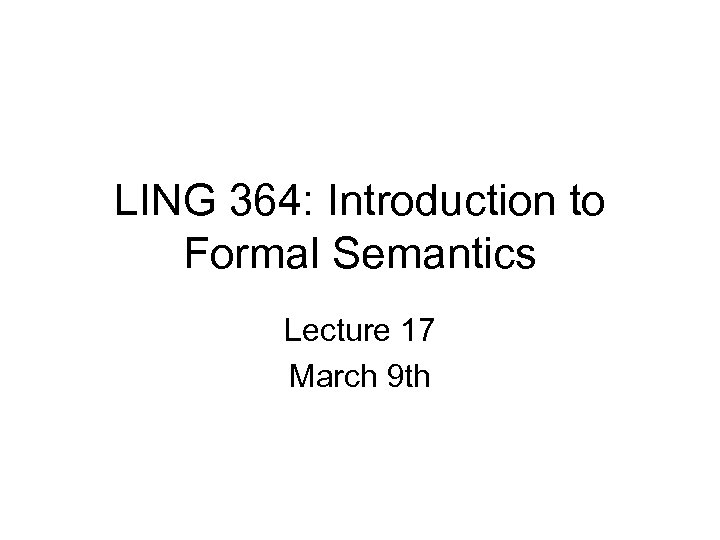LING 364: Introduction to Formal Semantics Lecture 17 March 9 thAdministrivia • No class next week – Have a good Spring break! – next lecture: 21 st March (in Comm 214)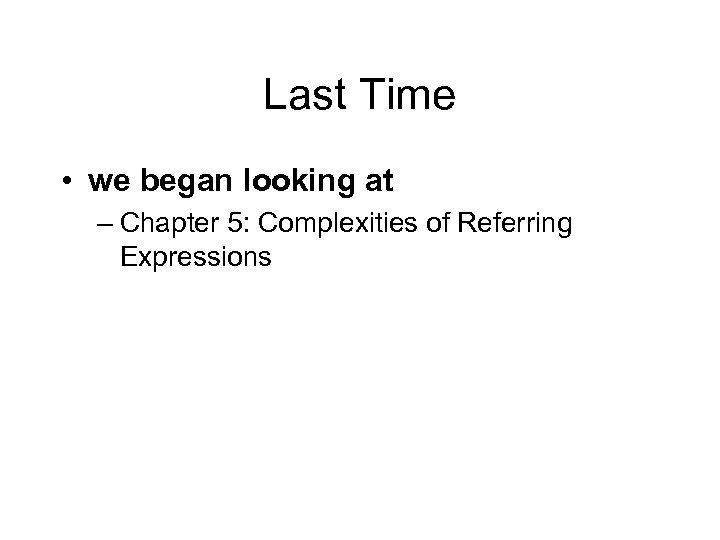Last Time • we began looking at – Chapter 5: Complexities of Referring ExpressionsToday’s Topic • Exercises for Chapter 5 • Quiz #4 at the end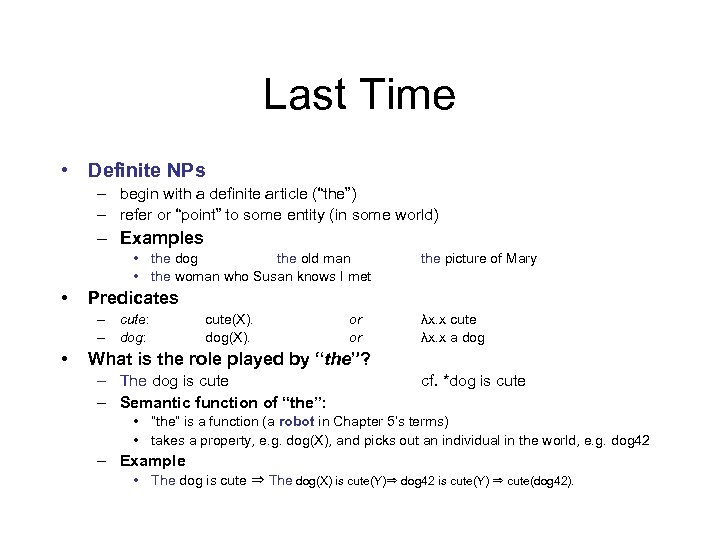Last Time • Definite NPs – begin with a definite article (“the”) – refer or “point” to some entity (in some world) – Examples • the dog the old man • the woman who Susan knows I met • Predicates – cute: – dog: • the picture of Mary cute(X). dog(X). or or λx. x cute λx. x a dog What is the role played by “the”? – The dog is cute – Semantic function of “the”: cf. *dog is cute • “the” is a function (a robot in Chapter 5’s terms) • takes a property, e. g. dog(X), and picks out an individual in the world, e. g. dog 42 – Example • The dog is cute ⇒ The dog(X) is cute(Y)⇒ dog 42 is cute(Y) ⇒ cute(dog 42).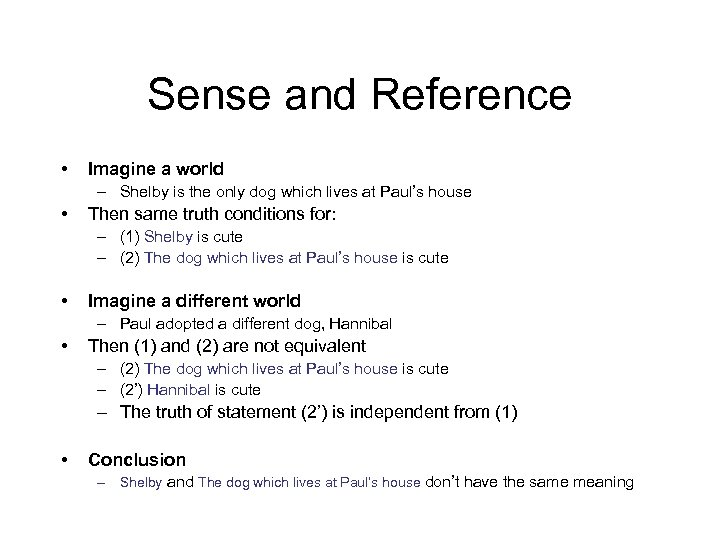Sense and Reference • Imagine a world – Shelby is the only dog which lives at Paul’s house • Then same truth conditions for: – (1) Shelby is cute – (2) The dog which lives at Paul’s house is cute • Imagine a different world – Paul adopted a different dog, Hannibal • Then (1) and (2) are not equivalent – (2) The dog which lives at Paul’s house is cute – (2’) Hannibal is cute – The truth of statement (2’) is independent from (1) • Conclusion – Shelby and The dog which lives at Paul’s house don’t have the same meaning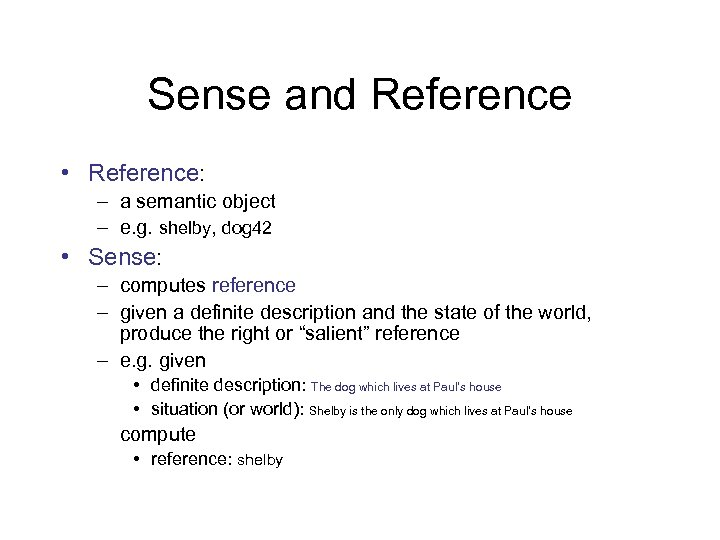Sense and Reference • Reference: – a semantic object – e. g. shelby, dog 42 • Sense: – computes reference – given a definite description and the state of the world, produce the right or “salient” reference – e. g. given • definite description: The dog which lives at Paul’s house • situation (or world): Shelby is the only dog which lives at Paul’s house compute • reference: shelby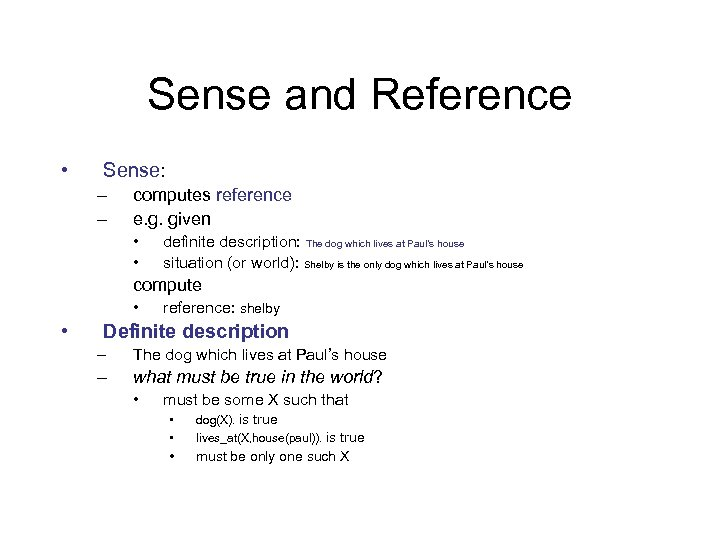Sense and Reference • Sense: – – computes reference e. g. given • • definite description: The dog which lives at Paul’s house situation (or world): Shelby is the only dog which lives at Paul’s house compute • • reference: shelby Definite description – The dog which lives at Paul’s house – what must be true in the world? • must be some X such that • • dog(X). is true • must be only one such X lives_at(X, house(paul)). is true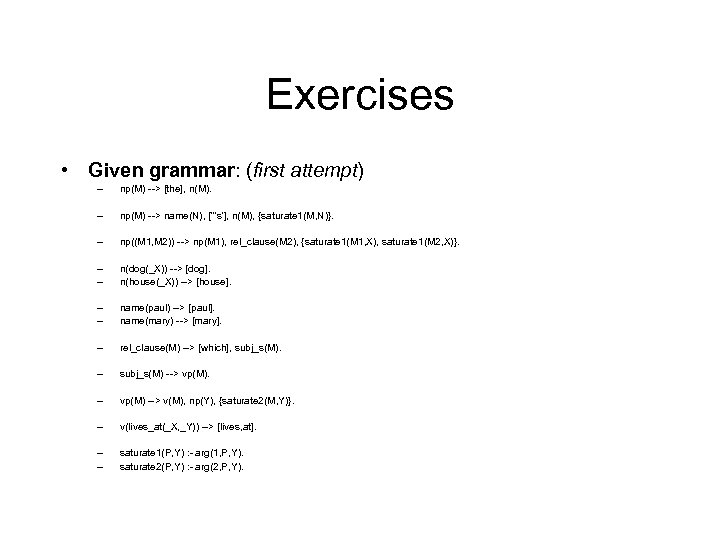Exercises • Given grammar: (first attempt) – np(M) --> [the], n(M). – np(M) --> name(N), ['''s'], n(M), {saturate 1(M, N)}. – np((M 1, M 2)) --> np(M 1), rel_clause(M 2), {saturate 1(M 1, X), saturate 1(M 2, X)}. – – n(dog(_X)) --> [dog]. n(house(_X)) --> [house]. – – name(paul) --> [paul]. name(mary) --> [mary]. – rel_clause(M) --> [which], subj_s(M). – subj_s(M) --> vp(M). – vp(M) --> v(M), np(Y), {saturate 2(M, Y)}. – v(lives_at(_X, _Y)) --> [lives, at]. – – saturate 1(P, Y) : - arg(1, P, Y). saturate 2(P, Y) : - arg(2, P, Y).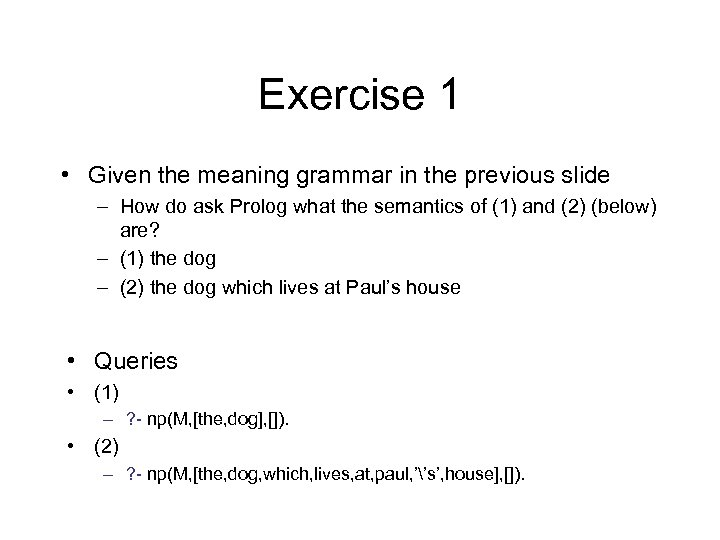Exercise 1 • Given the meaning grammar in the previous slide – How do ask Prolog what the semantics of (1) and (2) (below) are? – (1) the dog – (2) the dog which lives at Paul’s house • Queries • (1) – ? - np(M, [the, dog], []). • (2) – ? - np(M, [the, dog, which, lives, at, paul, ’’s’, house], []).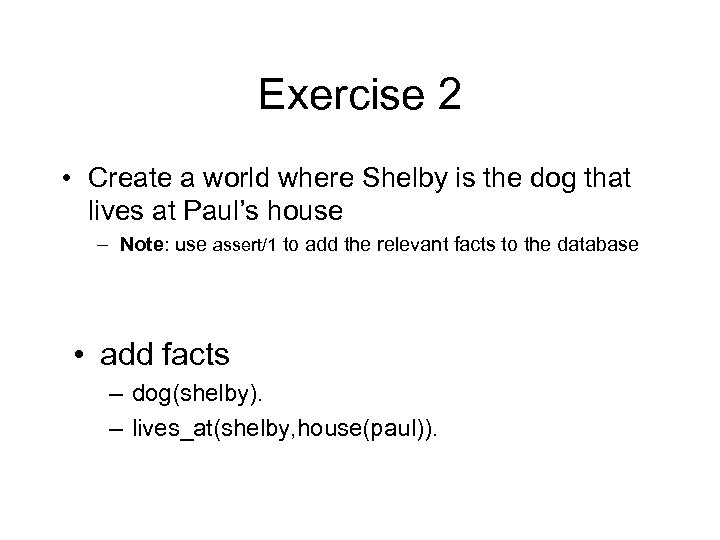Exercise 2 • Create a world where Shelby is the dog that lives at Paul’s house – Note: use assert/1 to add the relevant facts to the database • add facts – dog(shelby). – lives_at(shelby, house(paul)).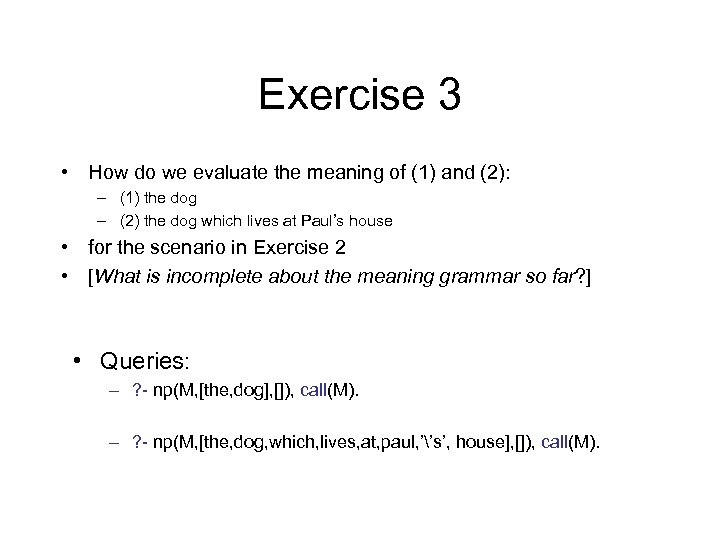Exercise 3 • How do we evaluate the meaning of (1) and (2): – (1) the dog – (2) the dog which lives at Paul’s house • for the scenario in Exercise 2 • [What is incomplete about the meaning grammar so far? ] • Queries: – ? - np(M, [the, dog], []), call(M). – ? - np(M, [the, dog, which, lives, at, paul, ’’s’, house], []), call(M).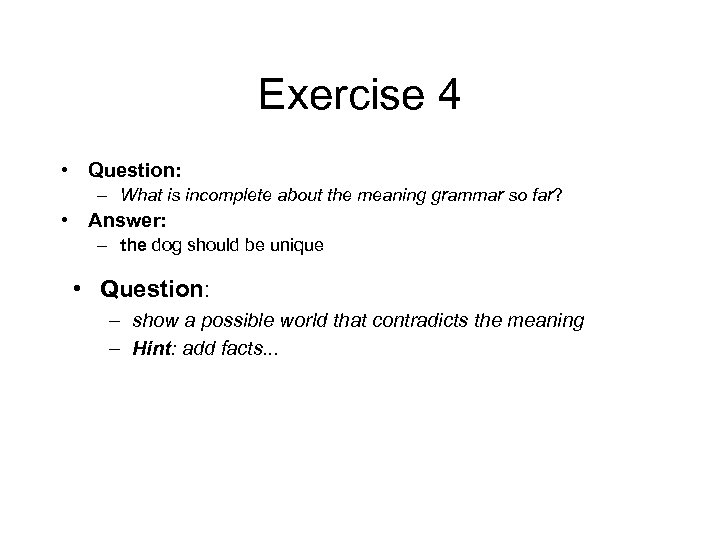Exercise 4 • Question: – What is incomplete about the meaning grammar so far? • Answer: – the dog should be unique • Question: – show a possible world that contradicts the meaning – Hint: add facts. . .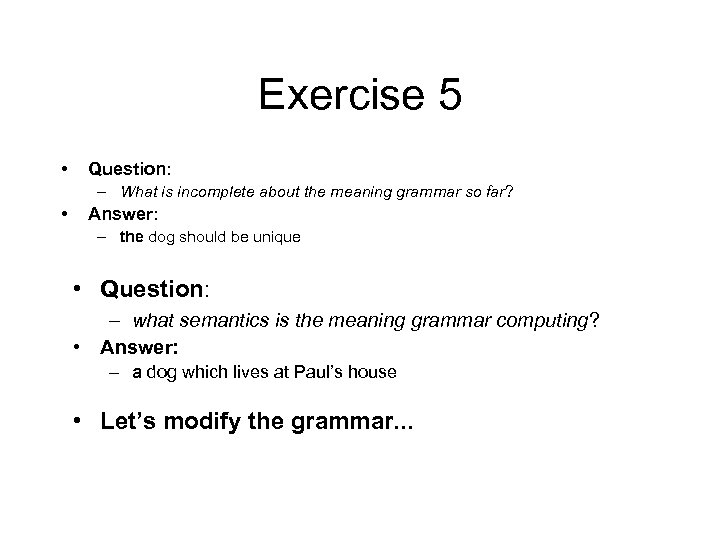Exercise 5 • Question: – What is incomplete about the meaning grammar so far? • Answer: – the dog should be unique • Question: – what semantics is the meaning grammar computing? • Answer: – a dog which lives at Paul’s house • Let’s modify the grammar. . .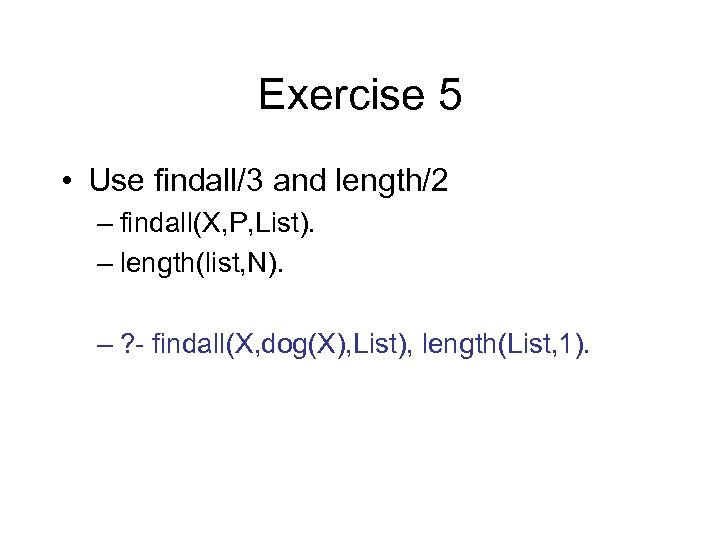Exercise 5 • Use findall/3 and length/2 – findall(X, P, List). – length(list, N). – ? - findall(X, dog(X), List), length(List, 1).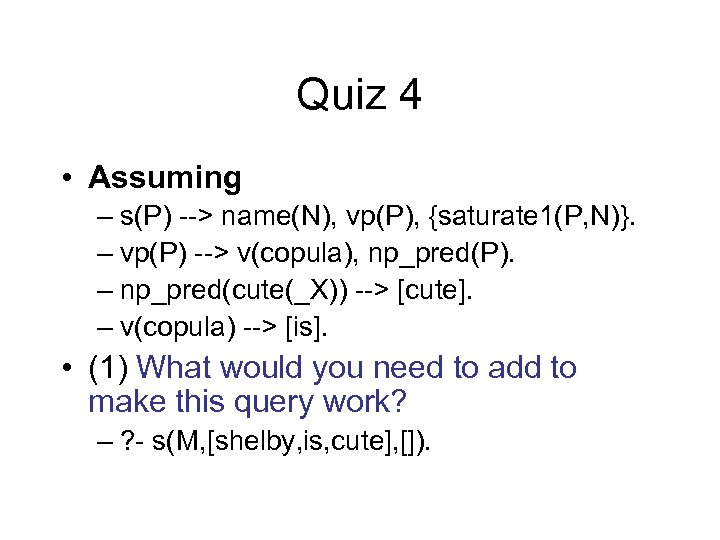Quiz 4 • Assuming – s(P) --> name(N), vp(P), {saturate 1(P, N)}. – vp(P) --> v(copula), np_pred(P). – np_pred(cute(_X)) --> [cute]. – v(copula) --> [is]. • (1) What would you need to add to make this query work? – ? - s(M, [shelby, is, cute], []).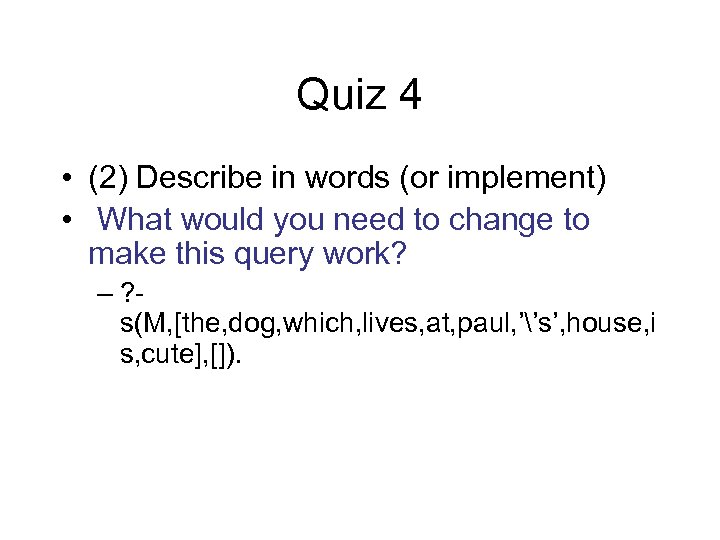Quiz 4 • (2) Describe in words (or implement) • What would you need to change to make this query work? – ? s(M, [the, dog, which, lives, at, paul, ’’s’, house, i s, cute], []).